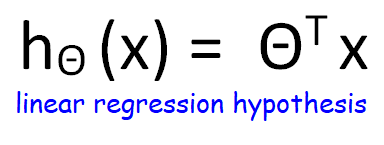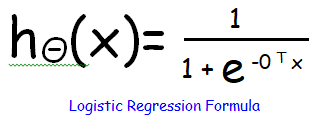# An easy way to understand linear classificationPosted by

### OutLine:

• What is a linear classification
• What is the difference between logistic and linear
• Logistic vs Linear  important points
• Python code
• Conclusion

# What is a linear classification?A linear classifier(L.C) is very similar to a logistic regressor, it classifies the data points based on the linear combination of features.

Now, what does it mean? The outcome of this classifier is derived from an equation that considers all the features during classification.

Therefore, LC separates the data samples using a line or a hyperplane in case of a higher dimension. Linear regression/classifiers both are part of the supervised machine learning model.

Hence, these two algorithms use labeled data for making predictions. Broadly there are three linear classifiers, those are perceptron, logistic regression, and support vector machines. In this blog, we shall keep our focus on logistic regression.

## What is logistic regression?

In the most simple terms, logistic regression is nothing but a linear regressor with a logistic function. Logistic regression is mainly used for binary classification.That means it separates between two different classes. However, LR is also used for multiclass classification problems. This logistic function, sigmoid in this case, divide the output of the function into two classes.The sigmoid function squeezes any input between 0 and 1. By default, the threshold value for the division of the function is 0.5. Therefore, if the outcome of the function is less than 0.5 then it is class A or class B.## Logistic vs Linear  important points:

1. Linear regressor tries to predict a continuous target variable (how much it will rain ?), whereas logistic regressor tries to predict a categorical variable(will it rain ?).
2. In linear regression, we try to fit a line to the closest to all the data points. Logistic regressor we try to divide the output into two classes using a sigmoid function.
3. R square, RMSE, MSE are used to measure the accuracy of the linear regressor. On the other hand, a confusion matrix is used to measure the accuracy of logistic regression.

## 5 assumptions of linear regression:

1. Linear relationship: The relationship between the independent and dependent variables has to be linear. It can be checked by plot a scatter graph.
2. Multivariate normality: A multivariate normal distribution is a vector in multiple normally distributed variables, such that any linear combination of the variables is also normally distributed.
3. No or little multicollinearity: independent variable that will be used in the linear regression should not have multicollinearity. Multi-colinearity happens when the independent variables are correlated.
4. No auto-correlation:  The random error components or disturbances are identically and independently distributed.
5. Homoscedasticity:  the error for all the data points are same along the best line of fit.

### Python code for logistic regression

You can download the data set from https://web.stanford.edu/~hastie/ElemStatLearn/.Look for “South African heart disease” data.

```import pandas as pd
import numpy as np
# taking out all the unique values
df['famhist'].unique()
# transforming the categorical variable to numerical variable
from sklearn.preprocessing import LabelEncoder
le=LabelEncoder()
le.fit(df['famhist'].unique())
df['famhist']=le.transform(df['famhist'])
# y is the target variable
y=df['chd']
# x hold all the independent features.
x=df.drop('chd',axis=1)
# scalling the independent features
from sklearn.preprocessing import StandardScaler
scaler = StandardScaler()
scaler.fit(x)
x=scaler.transform(x)
# building model to classify
from sklearn.linear_model import LogisticRegression
LR = LogisticRegression(random_state=0, solver='lbfgs', multi_class='ovr').fit(x, y)
# we are taking two rows to predict the class
LR.predict(x[350:352,:])

```

### Conclusion

In this blog, we discussed linear and logistic regression. We also discussed the sigmoid function and how it integrated with linear regression. The python code above is a small example that uses logistic regression for classification using a sigmoid function. In my upcoming blogs, we shall discuss more activation functions, perceptron, SVM. I hope, this blog has a clear idea of what is going on in logistic regression.

1.erotik izle says:

Thanks a lot for the article post. Really looking forward to read more. Keep writing. Leonore Allin Kyd

2.derma prime plus reviews says:

derma prime plus reviews
I was wondering if you ever thought of changing
the layout of your website? Its very well written; I love what youve got
to say. But maybe you could a little more in the way
of content so people could connect with it better.
Youve got an awful lot of text for only having 1 or 2 images.
Maybe you could space it out better?https://linktr.ee/DermaPrimePlusReviews